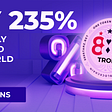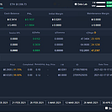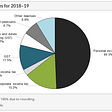# I.Similarities and differences Between Auto DDH and Advanced Auto DDH

## 4. Selection of parameters

• - If the current Delta is -2 BTC, then the Positive Deviation Delta is 1 BTC and the Negative Deviation Delta is 0.
• - If the current Delta is -4 BTC, then the Negative Deviation Delta is 1 BTC and the Positive Deviation Delta is 0.
• If you want the direction to be strictly neutral, put 0 here.
• If you want the overall Delta position to be long, e.g. 5 BTC long, put 5 here.
• If you want the overall Delta position to be short, e.g. 3 BTC short, put -3 here (i.e. minus 3).
• A long hedge is triggered when the Delta Target< total position Delta and the Positive Deviation Threshold exceeds the Positive Delta Threshold.
• A short hedge is triggered when the Delta Target > total position Delta and the Negative Deviation Threshold exceeds the Negative Delta Threshold.
• A hedging ratio of less than 100% means that the hedged amount is less than the Deviation Delta, and the minimum ratio needs to be greater than 0.
• A hedging ratio of more than 100% means that the hedged amount is greater than the Deviation Delta, and the maximum ratio should be no more than 150%.

# II.Cases

## Case 1: A hedging ratio of 100%

• When the total Delta rises to -2฿, the Positive Deviation Delta is 1฿, triggering the Positive Delta Threshold, and the long contract is hedged once. The total Delta returns to the Delta Target of -3฿ after the hedge is completed.
• When the total Delta drops to -3.5฿, the Negative Deviation Delta is 0.5฿, triggering the Negative Delta Threshold, and the short contract is hedged once. The total Delta also returns to the Delta Target of -3฿ after the hedge is completed.

## Case 2: A hedging ratio of 50%

• When the total Delta rises to -2฿, the Positive Deviation Delta is 1฿, triggering the Positive Delta Threshold, and the long contract is hedged once. The total Delta becomes -2.5฿ after the hedge is completed.
• When the total Delta drops to -3.5฿, the Negative Deviation Delta is 0.5฿, triggering the Negative Delta Threshold, and the short contract is hedged once. The total Delta becomes -3.35฿ after the hedge is completed.

## Case 3: A hedging ratio of 150%

• When the total Delta rises to -2฿, the Positive Deviation Delta is 1฿, triggering the Positive Delta Threshold, and the long contract is hedged once. The total Delta becomes -3.5฿ after the first over-hedge is completed. The Negative Deviation Delta now is 0.5฿, triggering Negative Delta Threshold, and the short contract is hedge once. After the second over-hedge is completed, the total Delta becomes -2.75฿.
• When the total Delta drops to -3.5฿, the Negative Deviation Delta is 0.5฿, triggering the Negative Delta Threshold, and the short contract is hedged once. The total Delta becomes -2.75฿ after the hedge is completed.

--

--

--

## More from Greeks.live

Love podcasts or audiobooks? Learn on the go with our new app.

## KuChain/Kratos AMA with Pool-X Full Transcript## How to Mint Crypto Papayas?## Orion Money## Yes, Cryptocurrencies Are Still Worthy Investments## Tokenomics 101## Anchor Protocol — Gold Standard for Passive income on Blockchain## How to Long Options PART 2 || Options Guide## Capital Gains Tax Changing## With the COVID variant spreading globally, the BTC market has finally hit rock bottom?